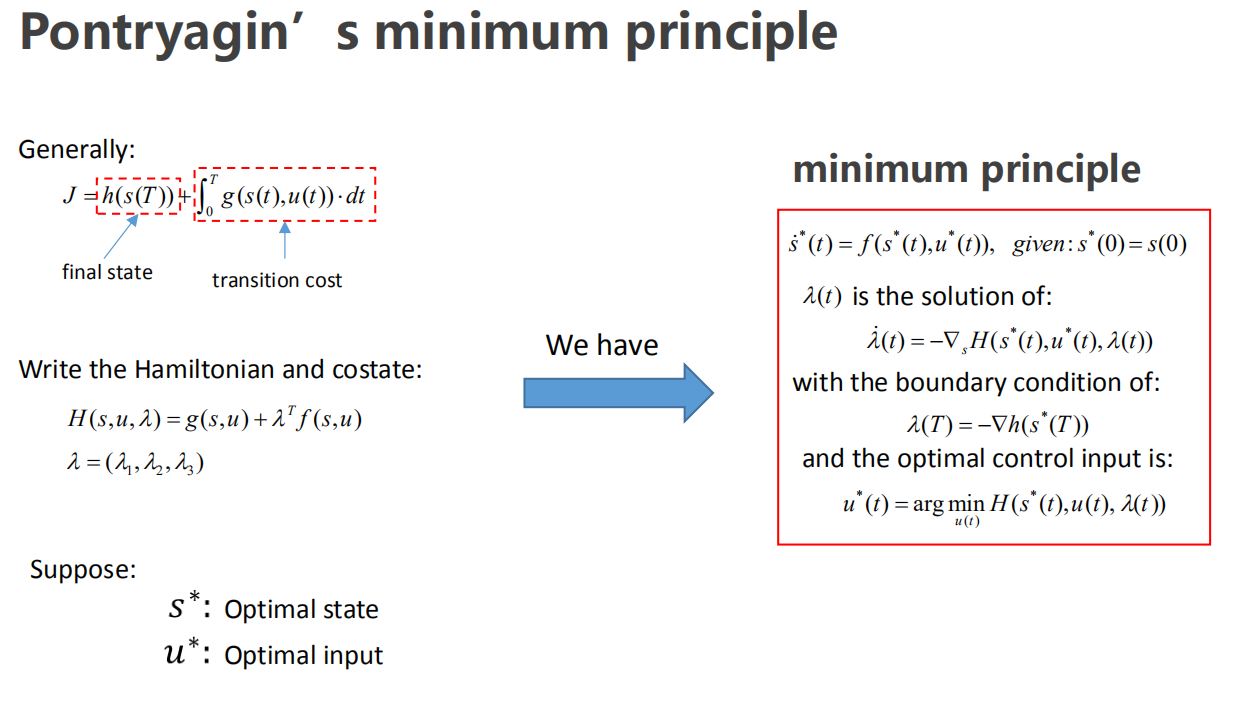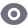# 用庞特里亚金极小值原理求解二阶系统的最优控制问题# 1. 问题描述

\begin{aligned} \dot { s } & = \left[ \begin{array} { l l } { 0 } & { 1 } \\ { 0 } & { 0 } \end{array} \right] s + \left[ \begin{array} { l } { 0 } \\ { 1 } \end{array} \right] u = \left[ \begin{array} { l l } { 0 } & { 1 } \\ { 0 } & { 0 } \end{array} \right] \left[ \begin{array} { l } { x } \\ \dot { x } \end{array} \right] + \left[ \begin{array} { l } { 0 } \\ { 1 } \end{array} \right] \ddot { x } \end{aligned}

$J = \frac { 1 } { 2 } \int _ { 0 } ^ { 2 } u ^ { 2 } d t$

# 2. 求解协态方程

$\dot { \lambda } ( t ) = - \nabla _ { s } H \left( s ^ { * } ( t ) , u ^ { * } ( t ) , \lambda ( t ) \right)$

$H = \frac { 1 } { 2 } u ^ { 2 } + \lambda _ { 1 } s_ { 2 } + \lambda _ { 2 } u$

$\dot { \lambda } _ { 1 } ( t ) = - \frac { \partial H } { \partial s _ { 1 } } = 0 \quad \Rightarrow \lambda _ { 1 } ( t ) = a _ { 1 }$

$\dot { \lambda } _ { 2 } ( t ) = - \frac { \partial H } { \partial s _ { 2 } } = - \lambda _ { 1 } ( t ) \Rightarrow \lambda _ { 2 } ( t ) = - a _ { 1 } t + a _ { 2 }$

# 3. 求解最优控制

$u ^ { * } ( t ) = \arg \min _ { u ( t ) } H \left( s ^ { * } ( t ) , u ( t ) , \lambda ( t ) \right)$

$\frac { \partial H } { \partial u } = u + \lambda _ { 2 } = 0 \quad \Rightarrow u = - \lambda _ { 2 } = a _ { 1 } t - a _ { 2 }$

$\begin{array} { l } {s _ { 1 } = \frac { 1 } { 6 } a _ { 1 } t ^ { 3 } - \frac { 1 } { 2 } a _ { 2 } t ^ { 2 } + a _ { 3 } t + a _ { 4 } } \\ { s _ { 2 } = \frac { 1 } { 2 } a _ { 1 } t ^ { 2 } - a _ { 2 } t + a _ { 3 } } \end{array}$

$u ^ { * } ( t ) = \frac { 9 } { 2 } t - 5$10-038447

#### 线性二次型最优控制器LQR设计原理以及matlab实现

02-2540万+

#### 推荐10个堪称神器的学习网站

09-128753

#### 消灭 Java 代码的“坏味道”

02-199万+

#### @程序员：GitHub这个项目快薅羊毛©️2020 CSDN 皮肤主题: 护眼 设计师: 闪电赇点击重新获取扫码支付1.余额是钱包充值的虚拟货币，按照1:1的比例进行支付金额的抵扣。
2.余额无法直接购买下载，可以购买VIP、C币套餐、付费专栏及课程。余额充值